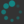## Problems

1. Finish filling in the multiplication table for the symmetry group of the triangle (this group is called $$\Dihed 3$$). You can use the widget to help you.
$\circ$ $e$ $r$ $R$ $f_1$ $f_2$ $f_3$
$e$
$r$ $f_3$
$R$
$f_1$ $f_2$
$f_2$ $R$
$f_3$

## Vistas

### Classification of finite simple groups

For a natural number $n,$ there is a finite (although possibly very large) number of groups of order $n.$ One can try to classify all the finite groups: this means, for each $n,$ giving a list of all the groups of order $n,$ or at least having a fairly explicit description of them. One can make some progress towards this goal: if $p$ is a prime number then the only group of order $p$ is the "rotational" group $\Cycl p,$ while the two different groups of order 6 we encountered are the only ones possible.

In general, however, this problem is not tractable: there are just way too many possibilities to get a handle on. There is a useful analogy to be made with chemistry: there are too many possible molecules for us to easily list them all. However, molecules are made up of atoms, and we can classify all the atoms: this is the periodic table of the elements. The problem is then reduced to understanding, for any given fixed collection of atoms, the different ways they can combine to form a molecule.

In group theory, one can break complicated groups up into smaller groups; groups that can't be broken down any further are called simple groups. The name "simple" does not mean that they are easy to understand, just that they can't be broken down any further—they are usually very complicated. Thus simple groups are the "atoms", while general groups are the "molecules". The "atoms" making up a group are called its composition factors. In the video, we encountered two groups of order 6: the symmetry group $$\Dihed 3$$ of the triangle, and the symmetry group $$\Cycl 6$$ of the colored hexagon. These have the same composition factors, namely a $$\Cycl 2$$ and a $$\Cycl 3,$$ but these building blocks are put together in different ways. Thus one can separate the problem of classifying groups in to two parts.

1. Determine all the finite simple groups; this can be thought of as the "periodic table" of groups.
2. Given any set of finite simple groups, determine the ways they can combine to form a group.

Both of these problems are very hard. However, after much effort the first step was completed in 2004. This is a major achievement of twentieth-century mathematics. The finite simple groups can be organized into three infinite families, as well as 27 "sporadic" outliers. These sporadic groups have mysterious connections to number theory and physics.